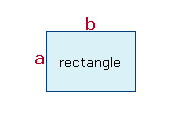# Fish Tank Calculator

You can use this useful fish tank calculator to determine key aspects of a fish tank; for example, the water surface area, the capacity, and the glass surface area. Simply choose the unit of measurement, input details of the base shape of the tank from the drop-down menu, input the dimensions identified a, b, c, input the height of the tank, and click on the "Calculate" button.

Aquarium Volume & Surface Area Calculator

ResultsTank Capacity (gallons):
Tank Capacity (liters):
Water Surface Area:
Glass Surface Area:

## Calculating the Volume and Surface Area of a Fish Tank

The surface area and volume of a fish tank are directly influenced by its dimensions and shape. To determine the capacity of an aquarium, it is important to take into consideration the amount of water that is displaced by the gravel and sand that is in the tank.

## Determine the Water Surface Area of a Fish Tank

There is a direct correlation between the amount of oxygen your fish have access to and the water surface area. The larger the surface area of a tank, the more fish you can place within it. If the tank is a regular rectangular shape, you can measure its surface area by multiplying the width by the length. If the tank is circular, the surface area can be computed by determining π/4 multiplied by the diameter squared. Our handy calculator can be particularly handy if you have an irregular-shaped aquarium.

Always take the measurements from inside the tank. If you take the measurements from the outside, they will include the thickness of the glass and, as such, will be inaccurate.

## Calculating Aquarium Volume

The capacity of a fish tank is the primary limiting factor in terms of how many fish you can have within it. You can determine the volume of an empty aquarium by multiplying the surface area of the water by the height of the tank. Again, you should make sure that you take the measurements from the tank interior to avoid including the base thickness.

To determine the capacity of your fish tank when it has gravel or rocks in it, you need to calculate the volume of water that is displaced by the rocks and then subtract this from the full volume. The volume of displacement varies according to the surface area of the base, the height of the gravel layer, and the coarseness of the material. If the base area is denoted by A and the gravel height is denoted by H, the volume of the gravel and rocks can be computed using one of the following formulas:

Volume ≈ 0.6*A*H   (Coarse gravel)

Volume ≈ 0.75*A*H   (Medium gravel)

Volume ≈ 0.9*A*H   (Fine gravel)

## Calculating the Glass Surface Area

It is common for algae to grow on the interior of an aquarium. If you are aware of the surface area of the glass, you are better placed to control algae growth and enable your fish to thrive. The glass area can be computed by multiplying the height of the tank by the inside perimeter.

If the tank is rectangular, the perimeter is 2*(W+L), where W is the width and L is the length. If the tank is circular, the perimeter is π multiplied by the diameter, or 2π multiplied by the radius.